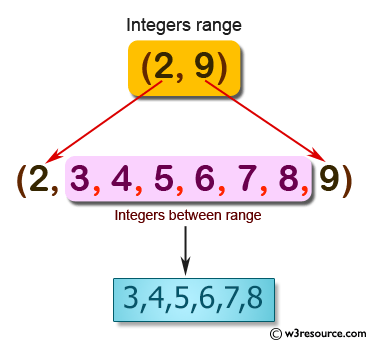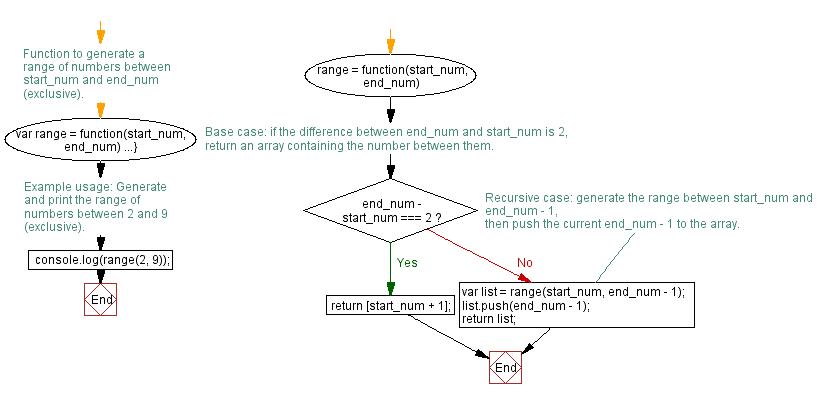# JavaScript: Get the integers in a range

## JavaScript Function: Exercise-3 with Solution

Write a JavaScript program to get the integers in range (x, y).

Example : range(2, 9)
Expected Output : [3, 4, 5, 6, 7, 8]

Pictorial Presentation:Sample Solution:-

HTML Code:

``````<!DOCTYPE html>
<html>
<meta charset="utf-8">
<title>Gets the integers from x to y</title>
<body>

</body>
</html>
```
```

JavaScript Code:

``````var range = function(start_num, end_num)
{
if (end_num - start_num === 2)
{
return [start_num + 1];
}
else
{
var list = range(start_num, end_num - 1);
list.push(end_num - 1);
return list;
}
};

console.log(range(2,9));
```
```

Output:

```[3,4,5,6,7,8]
```

Flowchart:Live Demo:

See the Pen javascript-recursion-function-exercise-3 by w3resource (@w3resource) on CodePen.

Improve this sample solution and post your code through Disqus

What is the difficulty level of this exercise?

Test your Programming skills with w3resource's quiz.

﻿

## JavaScript: Tips of the Day

Returns the difference (in days) between two dates

Example:

```const tips_DiffBetweenDates = (dateInitial, dateFinal) =>
(dateFinal - dateInitial) / (1000 * 3600 * 24);
console.log(tips_DiffBetweenDates(new Date('2020-05-13'), new Date('2020-06-05')));
```

Output:

```23
```